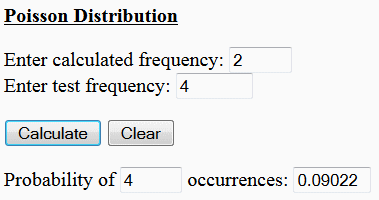# Poisson Distribution Calculator

The calculator that you can find at the bottom of this page is an online Poisson distribution calculator. It gives the probability of an event occurring a given number of times. You must know the expected frequency of the event.

We're just coding this formula:

probability = e[-f + x ln(f) - ln(x!)]

where

f = calculated frequency
x = test frequency

Example:

A firmware is being embedded in a a lot of 2000 microcontrollers. The probability of any microcontroller failing is 0.001 (just a conjecture and nothing to do with reality, ok?) Then we can expect 2 microcontrollers will suffer a severe problem (2000 x 0.001 = 2). What is the probability that 4 micros will fail?

We use our spreadsheet below and enter known data as input:

Enter calculated frequency = 2
Enter test frequency = 4

Probability of  4
occurrences =  0.09022

You'd have to enter the numbers like this...Please enable JavaScript codes, otherwise this tool won't work.

 Poisson Distribution Enter calculated frequency: Enter test frequency: Probability of occurrences:

Reference:
Poole, L.; Borchers, M.; Some Common Basic Programs; Osborne/McGraw-Hill;
3rd. edition; Berkeley, CA; 1979.

From 'Poisson Distribution Calculator' to home

From 'Poisson Distribution Calculator' to Free Online Calculators